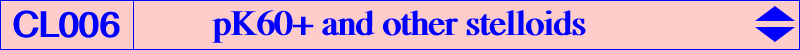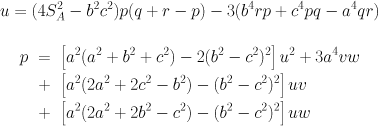pK60+ The pK with pole W = (p : q : r) and pivot P = (u : v : w) is a pK60+ if and only if P lies on the Neuberg cubic K001. Its pole W lies on the cubic K095 and its isopivot Q lies on K060. The locus of the common point X of the asymptotes is the bicircular quartic Q004. The correspondences between W and P are given by the formulas :Remark 1 : when P is one of the Fermat points X(13) or X(14), the pK60+ decomposes into the cevian lines of P. The corresponding poles are the barycentric squares X(11080), X(11085) of X(13), X(14) respectively. Remark 2 : the hessian cubic of a pK60+ is always a focal cubic with singular focus the common point X of the three asymptotes. The table below shows a selection of these cubics and other related points. Recall that W is the barycentric product P x Q.W on K095 P on K001 Q on K060 X on Q004 cubic hessian or centers on the hessian X(6) X(3) X(4) X(2) McCay K003 McCay hessian cubic K048 X(53) X(4) X(5) X(51) McCay orthic K049 McCay orthic hessian cubic K957 X(395) X(617) X(34295) X(619) Fermat K046b X(533), X(619) X(396) X(616) X(34296) X(618) Fermat K046a X(532), X(618) X(1989) X(30) X(5627) X(476) Tixier K037 Tixier hessian cubic K958 X(1990) X(5667) X(34297) X(14847) K543 X(30), X(14847) X(2160) X(1) X(79) X(14844) K097 X(14844) X(2161) X(3465) X(34299) X(34309) X(11063) X(399) X(14451) X(34306) X(11069) X(484) X(14452) X(34307) X(11070) X(1138) X(30) X(3258) Fermat K515 X(3258) X(11071) X(1263) X(1141) X(34308) X(11072) X(1276) ? ? X(11073) X(1277) ? ? X(11074) X(2132) ? ? X(11075) X(3065) X(80) X(34311) X(11076) X(3464) ? ? X(11077) X(3484) ? ? X(11079) X(74) X(265) X(34310) X(11080) X(13) X(13) X(13) see Remark 1 X(11081) X(5668) ? ? X(11082) X(8175) ? ? X(11083) X(15) X(11581) ? X(61) X(11084) X(1337) X(14373) ? X(11085) X(14) X(14) X(14) see Remark 1 X(11086) X(5669) ? ? X(11087) X(8174) ? ? X(11088) X(16) X(11582) ? X(62) X(11089) X(1338) X(14372) ? ? X(2133) X(34298) ? ? X(3440) X(621) ? ? X(3441) X(622) ? ? X(8487) X(1117) ? ? X(8431) X(6761) ? ? X(8494) X(11584) ? ? X(8446) X(11600) ? ? X(8456) X(11601) ? ? X(3466) X(34300) ? ? X(7164) X(34301) ? ? X(1157) X(34302) ? ? X(3483) X(34303) ? ? X(8439) X(34304) ? ? X(7165) X(34305) ?Other stelloids The following table gathers together all listed stelloids with their respective types and their listed isogonal transforms (if any) in the last column. The orange (resp. light blue) cells correspond to stelloids having the same asymptotic directions as K003 (resp. K024). Their isogonal transforms are CircumNormal (resp. CircumTangential) cubics as in Table 25. The "orange" cubics are called McCay stelloids when they are circum-cubics and then, they are spK(X3, Q) for some Q. The asymptotes concur at X such that QX = 1/3 QH (vectors). See here for further properties. Notations : o, c, n denote a circumscribed, central, nodal cubic respectively.stelloid X = X(i) o c n type X(i) on the curve for i = stelloid* K003 2 o pK 1, 3, 4, 1075, 1745, 3362, 13855, 39641, 39642, 46357, 46358 K003 K024 2 o nK0 K024 K026 5 o c psK, spK 3, 4, 5, 5403, 5404, 8798, 14363 K361 K028 381 o n psK, spK 3, 4, 8, 76, 847, 3557, 3558, 3730, 8743, 10571, 14246, 14247, 14248, 14249, 14250, 14251, 14252, 14253, 14254, 14255, 14256, 14257, 14258, 14259, 14260, 14261, 14262, 14263, 14264, 14265, 14266, 14267, 14268, 25043, 34243, 34756, 38539, 38936, 38937, 38938, 38939, 39263, 39264, 39265, 39267, 39268, 39269, 39270 K009 K037 476 o pK 30, 5627 K374 K046a 618 o c pK 13, 616, 618, 34296 K046b 619 o c pK 14, 617, 619, 34295 K049 51 o pK 4, 5, 52, 847, 39641, 39642 K373 K054 13364 o n spK 4, 5, 143, 14254, 14374, 14375, 14584, 14632, 14633, 22100, 27359, 27375, 34356, 34434 K071 5891 o psK, spK 4, 5, 20, 76, 5562, 15318 K077 376 1, 3, 20, 170, 194, 7991 K078 3524 1, 2, 3, 165, 5373, 6194, 21214, 32524 K080 3 o c spK 3, 4, 20, 1670, 1671, 15318 K405 K094 599 o nK K097 14844 o pK 1, 79 K100 3 c 1, 3, 40, 1670, 1671, 17749 K115 3060 o spK0 4, 6243, 25043, 39641, 39642 K139 5627 o c nK 30, 5627 K204 34365 o nK0 K205 14846 o nK0 K213 2 o c nK 2, 11058 K230 14629 o n cK 80, 2222, 23838, 37630 K258 549 n 1, 3, 5, 39, 2140, 14823, 15345 K268 3819 o spK0 4, 20, 140, 15318, 15644, 39641, 39642 K309 5054 o spK 3, 4, 376, 1340, 1341, 39158, 39159, 39160, 39161 K358 3545 o spK 3, 4, 381, 39162, 39163, 39164, 39165 K412 14845 o spK 2, 4, 5, 51, 262, 14249 K513 14846 o 6, 15, 16, 74, 265, 3016 K514 6781 o spK 4, 15, 16, 39, 6781 K515 3258 o pK 30, 1138, 31378 K516 262 o spK0 4, 3095, 14251, 39641, 39642 K525 4 o c spK 3, 4, 382 K543 14847 o pK 5667, 34297 K580 568 o spK 4, 847, 5889 K581 5055 o spK 2, 3, 4, 262 K582 14848 o spK 2, 4, 6, 262, 4846, 14246, 20423, 31861 K594 5603 o n spK 1, 4, 1482, 14260, 17753 K595 14849 o n 74, 98, 265, 290, 671, 9140 K596 14850 o n 74, 99, 265, 290, 34174 K597 14851 o n 30, 74, 265, 477, 43707 K598 6 K613 14643 o nK, spK 4, 110, 1113, 1114, 38936, 41512 K643 14561 o spK0 4, 6, 4846, 8743, 39641, 39642 K665 549 o spK 3, 4, 39, 550 K664 K669 14644 o nK, spK 4, 74, 265, 14254 K670 14852 o psK, spK 4, 26, 64, 68, 847 K708 14853 o spK0 4, 1344, 1345, 1351, 14248, 18906, 39641, 39642 K714 14640 o spK 4, 14374, 14375, 30493 K724 14854 o n psK 74, 265, 5961, 6344, 11060, 39373, 39374, 39375, 39376 K827 14855 o spK 4, 20, 550, 15318 K833 2 c 2, 3, 381, 39162, 39163, 39164, 39165, 39641, 39642 K852 376 o spK 3, 4, 1657, 42411, 42412 K910 140 1, 3, 546, 1385 K911 5054 1, 3, 381, 1340, 1341, 3576, 39162, 39163, 39164, 39165 K928 10606 o spK 4, 20, 64, 68, 12084, 15318, 20427, 38937 K929 15061 o 74, 265, 1113, 1114, 15328 K930 15362 o spK 2, 4, 23, 74, 262, 265, 15360, 15363 K931 K1063 5667 1, 3, 8, 40, 21214, 21227, 21228, 21229, 21306, 21307 K1064 21445 3, 15, 16, 98, 385, 21444 K1098 7709 3, 194, 39641, 39642 K1101 32447 o spK 4, 194, 14251 K1136 34290 o nK0 K1137 381 o nK K1139 5640 o spK 4, 568, 14254, 39641, 39642 K1140 5654 o psK, spK 4, 155, 34756, 34757 K1161 5085 3, 6, 40122 K1261 3 c 2, 3, 376, 1670, 1671, 7757, 33706 K1292 ? 1, 6, 20, 194, 35237, 46264Additional remarks : • See the related Table 54 for green cells. These are the spK(X3, Q on the Euler line). • the circum-cubics highlighted in yellow are those of Table 51 : they are spK(X3, Q on the Brocard axis). They form a pencil of K0s passing through the infinite points of K003, X(4) and the imaginary foci of the Brocard ellipse i.e. common points of the Brocard axis and the Kiepert hyperbola. The asymptotes concur at X on the line X(2), X(51), X(262), X(263), X(373), X(511), X(2979), X(3060), etc.